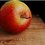# Graph of the World

I wanted to post this note many months ago, but I guess I procastinated.In this note, I would like to show how to do a similar graph like the above, to graph literally anything in a single function (As long as it is a function of x), and invite anybody to make a graph like this, depicting an object (be it a building, or an ant). Then, I will compile the graphs together such that graphing the single function depicts any object we have made.

In the image above, I have depicted the Empire State Building and the Singapore Parliament house in a single function.

$\textbf{How to do it}$

First, let me introduce this function: $\frac{\left|\left(x-k\right)-\left|x-k\right|\right|}{2\left(k-x\right)}+\frac{\left|\left(x+a\right)-\left|x+a\right|\right|}{2\left(x+a\right)}$

The above function, basically graphs $y=0$ from $x=-\infty \text{ to } x=-a$ and $x=k \text{ to } x=\infty$, and $y=1$ from $x=-a \text{ to } x=k$, assuming $a$ and $k$ to be positive real numbers.Now, if you can see where this is going, if I $\times$ that above function by $f(x)$, what would I get? I get $y=0$ from $x=-\infty \text{ to } x=-a$ and $x=k \text{ to } x=\infty$, and $y=f(x)$ from $x=-a \text{ to } x=k$.

For the case of $f(x) = x^{2}$, $a=2$ and $k=1$:

Graph of $x^2\left(\frac{\left|\left(x-1\right)-\left|x-1\right|\right|}{2\left(1-x\right)}+\frac{\left|\left(x+2\right)-\left|x+2\right|\right|}{2\left(x+2\right)}\right)$You can try it yourself here

Now, what if we want to graph $y=x^{2}$ from $x=-2$ to $x=1$ and then $y=(x-2)^{3}$ from $x=1$ to $x=3$? Easy, we just add $2$ functions together:

Graph of $x^2\left(\frac{\left|\left(x-1\right)-\left|x-1\right|\right|}{2\left(1-x\right)}+\frac{\left|\left(x+2\right)-\left|x+2\right|\right|}{2\left(x+2\right)}\right)+\left(x-2\right)^3\left(\frac{\left|\left(x-3\right)-\left|x-3\right|\right|}{2\left(3-x\right)}+\frac{\left|\left(x-1\right)-\left|x-1\right|\right|}{2\left(x-1\right)}\right)$And so we are done with the basis of how to graph any object you want, all you have to do is keep adding the functions together until you get the desired shape.

Now, what if your desired shape is referenced from a picture? No worries, Desmos enables you to add a picture for reference:Sidenote: To achieve shading (For the picture above, the shading is red), just add an inequality. To know what I mean, try $y>x$. The above picture took me about 45 minutes.

You might want to see this as an example

$\textbf{Graph Submission details}$

If you want to make one of these graphs, and want to submit yours too, just post the link of your graph into the comments section. However, for your graph to be accepted, it has to pass some requirements:

Requirements:

$\bullet$ Your graph must be $y=0$ from $x=-\infty$ to $x=k_{1}$ and from $x=\infty$ to $x=k_{2}$, where $k_{1}\le x\le k_{2}$ is the section where your object exists. This is to allow me to add multiple objects to a single function's graph (Like how I added the Empire State Building and the Singapore Parliament House together into a single function.)

$\bullet$ Your Graph should have a height of roughly between $5$ to $20$. This is to avoid graphs that are too small or too big to be added into the compilation.

Well that's about it, have fun making one of these graphs! If you have any questions, post them in the comments section.Note by Julian Poon
5 years, 11 months ago

This discussion board is a place to discuss our Daily Challenges and the math and science related to those challenges. Explanations are more than just a solution — they should explain the steps and thinking strategies that you used to obtain the solution. Comments should further the discussion of math and science.

When posting on Brilliant:

• Use the emojis to react to an explanation, whether you're congratulating a job well done , or just really confused .
• Ask specific questions about the challenge or the steps in somebody's explanation. Well-posed questions can add a lot to the discussion, but posting "I don't understand!" doesn't help anyone.
• Try to contribute something new to the discussion, whether it is an extension, generalization or other idea related to the challenge.
• Stay on topic — we're all here to learn more about math and science, not to hear about your favorite get-rich-quick scheme or current world events.

MarkdownAppears as
*italics* or _italics_ italics
**bold** or __bold__ bold
- bulleted- list
• bulleted
• list
1. numbered2. list
1. numbered
2. list
Note: you must add a full line of space before and after lists for them to show up correctly
paragraph 1paragraph 2

paragraph 1

paragraph 2

[example link](https://brilliant.org)example link
> This is a quote
This is a quote
    # I indented these lines
# 4 spaces, and now they show
# up as a code block.

print "hello world"
# I indented these lines
# 4 spaces, and now they show
# up as a code block.

print "hello world"
MathAppears as
Remember to wrap math in $$ ... $$ or $ ... $ to ensure proper formatting.
2 \times 3 $2 \times 3$
2^{34} $2^{34}$
a_{i-1} $a_{i-1}$
\frac{2}{3} $\frac{2}{3}$
\sqrt{2} $\sqrt{2}$
\sum_{i=1}^3 $\sum_{i=1}^3$
\sin \theta $\sin \theta$
\boxed{123} $\boxed{123}$

## Comments

Sort by:

Top Newest

In response to Apple: $\color{#FFFFFF}{\text{Excellent work!}}$

- 5 years, 11 months ago

Log in to reply

In response to Lameness: $\color{#FFFFFF}{\text{More Lameness}}$

- 5 years, 10 months ago

Log in to reply

$\color{#FFFFFF}{\text{I am not lame -\_-}}$

- 5 years, 10 months ago

Log in to reply

On a slightly more practical level, NURBS, or "non-uniform rational B-spline", have been developed to provide functions of fairly arbitrary curves and surfaces for computer graphics and industrial uses. They are extremely powerful and amendable to mathematical methods, as compared to conventional "elementary functions" of mathematics. What you are proposing is something analogous, but for discretized shapes. It's a start, but needs more work to attain the same functionality as NURBS. See Bezier Curves for an easy-to-understand text on a subset of NURBS.

- 5 years, 10 months ago

Log in to reply

Wow. I didn't know this kind of thing was practical

- 5 years, 10 months ago

Log in to reply

×

Problem Loading...

Note Loading...

Set Loading...